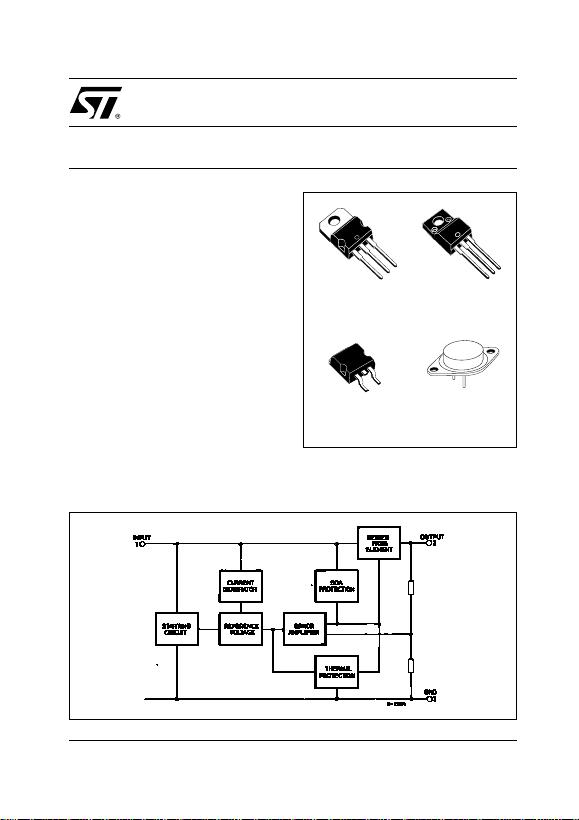1/34November 2004
OUTPUT CURRENT TO 1.5A
OU TPUT VOLTA GES OF 5; 5.2; 6; 8; 8.5; 9;
10; 12; 15; 18; 24V
THERMAL OVERLOAD PROTECTION
SHORT CIRCUIT PROTECTION
OUTPUT TRANSITION SOA PROTECTION
DESCRIPTION
The L7800 series of three-terminal positive
regulators is available in TO-220, TO-220FP,
TO-220FM, TO-3 and D2PAK packages and
several f ixed output voltages, making it useful i n a
wide range of applications. These regulators can
provide local on-card regulation, eliminating the
distribution proble ms associated with single point
regulation. Each type employs internal current
limiting, thermal shut-down and safe area
protection, making it essentially indestructible. If
adequate heat sinking is provided, they can
deliver over 1A ou tput current. Although des ign ed
primarily as fixed voltage regulators, these
dev ices c an b e us ed with ex ternal c om ponents t o
obtain adjust able voltage and currents.
L7800
SERIES
POSI TIVE VOL T AG E RE GULA TOR S
Fi gure 1: S ch ematic Di agra m
TO-220
D2PAK TO-3
TO-220FP
TO-220FM
Rev. 12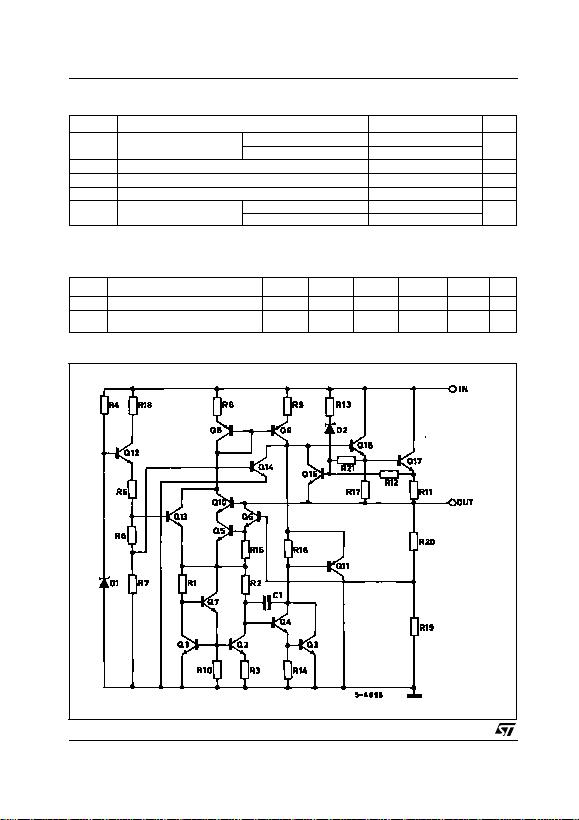L7800 SERIES
2/34
Table 1: Absolute Maximum Ratings
Absolute Maximum Ratings are those values beyond which damage to the device may occur. Functional operation under these condition is
not implie d.
Table 2: Therm al Data
Figure 2: Schematic Diagram
Symbol Parameter Value Unit
VIDC Input Voltage for VO= 5 to 18V 35 V
for VO= 20, 24V 40
IOOutput Current Internally Limited
Ptot Power Dissipation Internally Limited
Tstg Storage Temperature Range -65 to 150 °C
Top Operating Junction T emperature
Range for L7800 -55 to 150 °C
for L7800C 0 to 150
Symbol Parameter D2PAK TO-220 TO-220FP TO-220FM TO-3 Unit
Rthj-case Thermal Resistance Junction-case Max 355 54°C/W
Rthj-amb Thermal Resistance Junction-ambient
Max 62.5 50 60 60 35 °C/W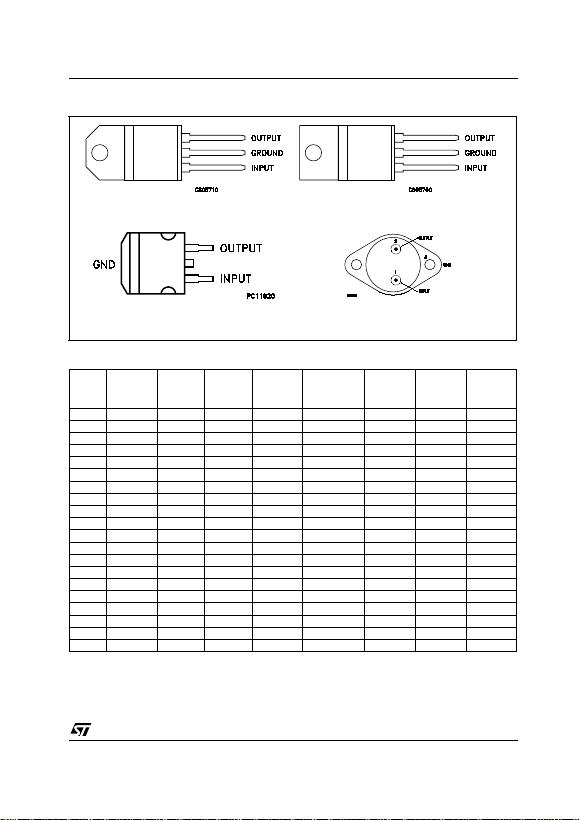L7800 SERIES
3/34
Fi gure 3: C onne ction Dia gra m (top view)
Table 3: Order Codes
(* ) A vailable in Tape & Reel wit h t he suffix " -T R".
TYPE TO-220
(A Type) TO-220
(C Type) TO-220
(E Type) D2PAK
(A Type) (*)
D2PAK
(C Type)
(T & R) TO-220FP TO-220FM TO-3
L7805 L7805T
L7805C L7805CV L7805C-V L7805CV1 L7805CD2T L7805C-D2TR L7805CP L7805CF L7805CT
L7852C L7852CV L7852CD2T L7852CP L7852CF L7852CT
L7806 L7806T
L7806C L7806CV L7806C-V L7806CD2T L7806CP L7806CF L7806CT
L7808 L7808T
L7808C L7808CV L7808C-V L7808CD2T L7808CP L7808CF L7808CT
L7885C L7885CV L7885CD2T L7885CP L7885CF L7885CT
L7809C L7809CV L7809C-V L7809CD2T L7809CP L7809CF L7809CT
L7810C L7810CV L7810CD2T L7810CP
L7812 L7812T
L7812C L7812CV L7812C-V L7812CD2T L7812CP L7812CF L7812CT
L7815 L7815T
L7815C L7815CV L7815C-V L7815CD2T L7815CP L7815CF L7815CT
L7818 L7818T
L7818C L7818CV L7818CD2T L7818CP L7818CF L7818CT
L7820 L7820T
L7820C L7820CV L7820CD2T L7820CP L7820CF L7820CT
L7824 L7824T
L7824C L7824CV L7824CD2T L7824CP L7824CF L7824CT
TO-220 (Any Type)
TO-3D2PA K ( A ny Ty p e )
TO-220FP/TO-220FM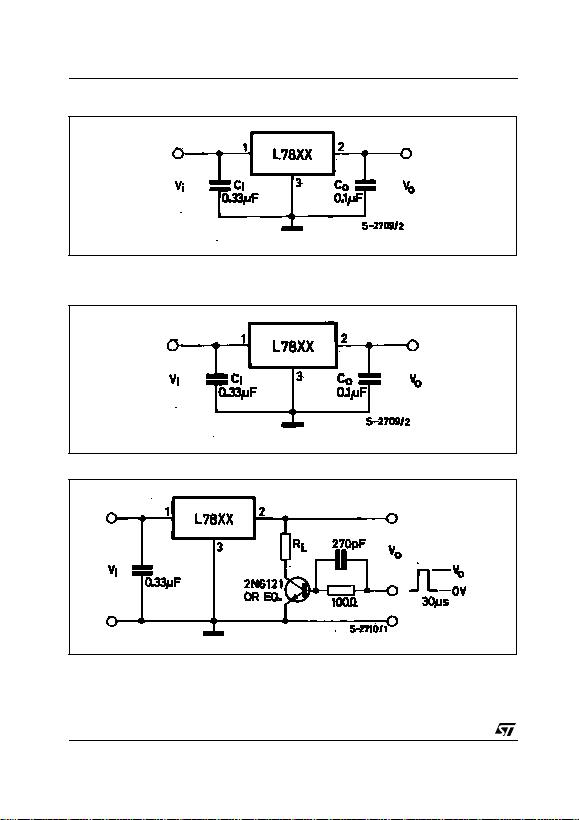L7800 SERIES
4/34
Fi gure 4: A pplication Ci rcuits
TEST CIRCUITS
Figure 5: DC Parameter
Fi gure 6: Loa d Re gulation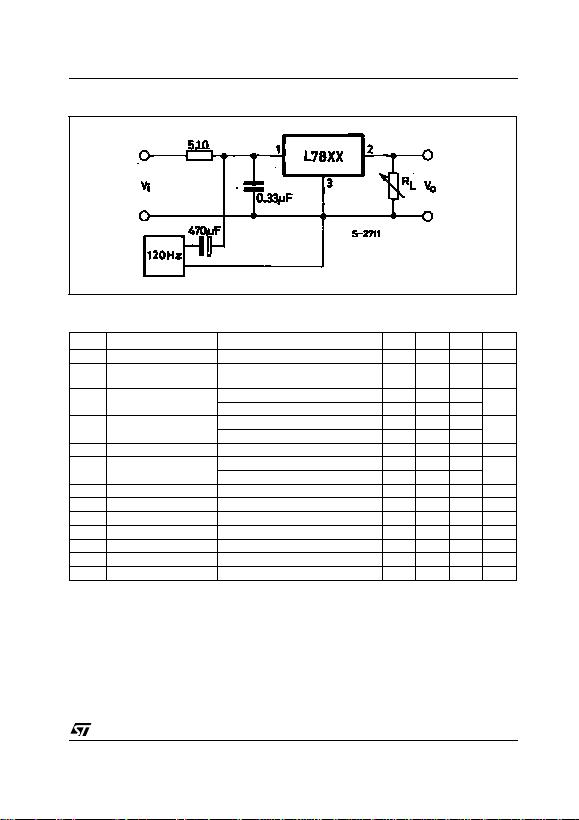L7800 SERIES
5/34
Fi gure 7: R ipple Rejec tion
Table 4: Electrical Characteristics Of L7805 (r e fe r to th e test circuits, TJ = -55 to 150°C, VI = 10V ,
IO = 500 mA, CI = 0.33 µF , CO = 0.1 µF unless otherwise specified).
(*) Load and line regulation are specified at constant junction temperature. Changes in VO due to heating effect s must be taken int o acc ount
separately. Pulse te sting with low duty cycle is used.
Symbol Parameter Test Conditions Min. Typ. Max. Unit
VOOutput Voltage TJ = 25°C 4.8 5 5.2 V
VOOutput Voltage IO = 5 mA to 1 A PO 15W
VI = 8 to 20 V 4.65 5 5.35 V
VO(*) Line Regulation VI = 7 to 25 V TJ = 25°C 3 50 mV
VI = 8 to 12 V TJ = 25°C 1 25
VO(*) Load Regulation IO = 5 mA to 1.5 A TJ = 25°C 100 mV
IO = 250 to 750 mA TJ = 25°C 25
IdQuiescent Current TJ = 25°C 6 mA
IdQuiescent Current Change IO = 5 mA to 1 A 0.5 mA
VI = 8 to 25 V 0.8
VO/T Output Voltage Drift IO = 5 mA 0.6 mV/°C
eN Output Noise Voltage B =10Hz to 100KHz TJ = 25°C 40 µV/VO
SVR Supply Voltage Rejection VI = 8 to 18 V f = 120Hz 68 dB
VdDropout Voltage IO = 1 A TJ = 25°C 2 2.5 V
ROOutput Resistance f = 1 KHz 17 m
Isc Short Circuit Current VI = 35 V TJ = 25°C 0.75 1.2 A
Iscp Short Circuit Peak Current TJ = 25°C 1.3 2.2 3.3 A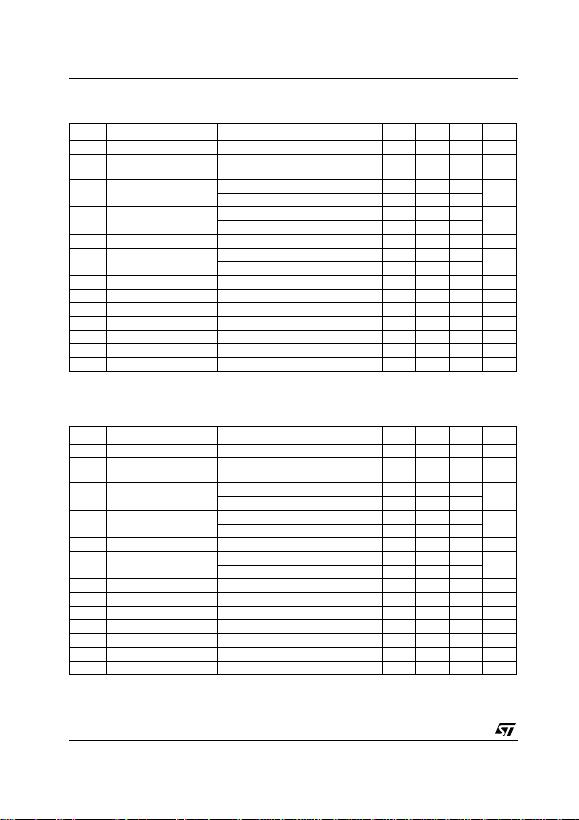L7800 SERIES
6/34
Table 5: Electrical Characteristics Of L7806 (r e fe r to th e test circuits, TJ = -55 to 150°C, VI = 11V ,
IO = 500 mA, CI = 0.33 µF , CO = 0.1 µF unless otherwise specified).
(*) Load and line regulation are specified at constant junction temperature. Changes in VO due to heating effect s must be taken int o acc ount
separately. Pulse te sting with low duty cycle is used.
Table 6: Electrical Characteristics Of L7808 (r e fe r to th e test circuits, TJ = -55 to 150°C, VI = 14V ,
IO = 500 mA, CI = 0.33 µF , CO = 0.1 µF unless otherwise specified).
(*) Load and line regulation are specified at constant junction temperature. Changes in VO due to heating effect s must be taken int o acc ount
separately. Pulse te sting with low duty cycle is used.
Symbol Parameter Test Conditions Min. Typ. Max. Unit
VOOutput Voltage TJ = 25°C 5.75 6 6.25 V
VOOutput Voltage IO = 5 mA to 1 A PO 15W
VI = 9 to 21 V 5.65 6 6.35 V
VO(*) Line Regulation VI = 8 to 25 V TJ = 25°C 60 mV
VI = 9 to 13 V TJ = 25°C 30
VO(*) Load Regulation IO = 5 mA to 1.5 A TJ = 25°C 100 mV
IO = 250 to 750 mA TJ = 25°C 30
IdQuiescent Current TJ = 25°C 6 mA
IdQuiescent Current Change IO = 5 mA to 1 A 0.5 mA
VI = 9 to 25 V 0.8
VO/T Output Voltage Drift IO = 5 mA 0.7 mV/°C
eN Output Noise Voltage B =10Hz to 100KHz TJ = 25°C 40 µV/VO
SVR Supply Voltage Rejection VI = 9 to 19 V f = 120Hz 65 dB
VdDropout Voltage IO = 1 A TJ = 25°C 2 2.5 V
ROOutput Resistance f = 1 KHz 19 m
Isc Short Circuit Current VI = 35 V TJ = 25°C 0.75 1.2 A
Iscp Short Circuit Peak Current TJ = 25°C 1.3 2.2 3.3 A
Symbol Parameter Test Conditions Min. Typ. Max. Unit
VOOutput Voltage TJ = 25°C 7.7 8 8.3 V
VOOutput Voltage IO = 5 mA to 1 A PO 15W
VI = 11.5 to 23 V 7.688.4V
VO(*) Line Regulation VI = 10.5 to 25 V T J = 25°C 80 mV
VI = 11 to 17 V TJ = 25°C 40
VO(*) Load Regulation IO = 5 mA to 1.5 A TJ = 25°C 100 mV
IO = 250 to 750 mA TJ = 25°C 40
IdQuiescent Current TJ = 25°C 6 mA
IdQuiescent Current Change IO = 5 mA to 1 A 0.5 mA
VI = 11.5 to 25 V 0.8
VO/T Output Voltage Drift IO = 5 mA 1 mV/°C
eN Output Noise Voltage B =10Hz to 100KHz TJ = 25°C 40 µV/VO
SVR Supply Voltage Rejection VI = 11.5 to 21.5 V f = 120Hz 62 dB
VdDropout Voltage IO = 1 A TJ = 25°C 2 2.5 V
ROOutput Resistance f = 1 KHz 16 m
Isc Short Circuit Current VI = 35 V TJ = 25°C 0.75 1.2 A
Iscp Short Circuit Peak Current TJ = 25°C 1.3 2.2 3.3 A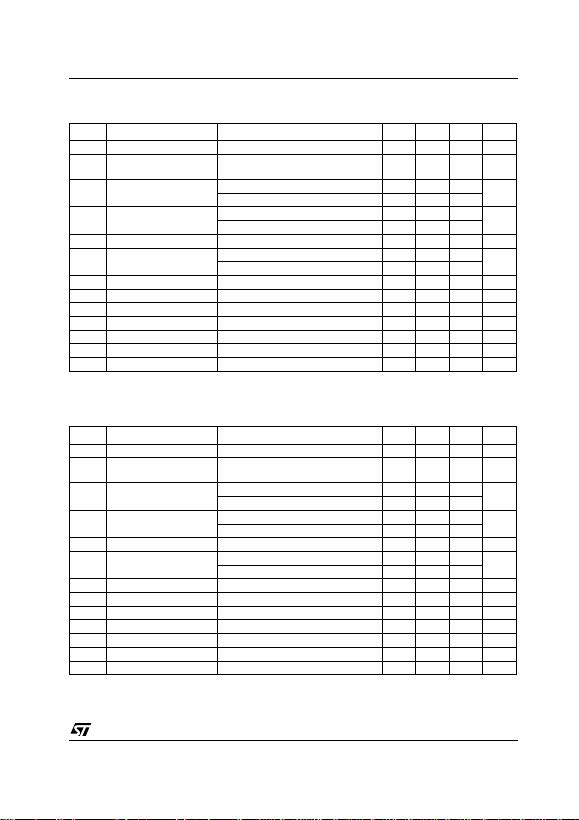L7800 SERIES
7/34
Table 7: Electrical Characteristics Of L7812 (r e fe r to th e test circuits, TJ = -55 to 150°C, VI = 19V ,
IO = 500 mA, CI = 0.33 µF , CO = 0.1 µF unless otherwise specified).
(*) Load and line regulation are specified at constant junction temperature. Changes in VO due to heating effect s must be taken int o acc ount
separately. Pulse te sting with low duty cycle is used.
Table 8: Electrical Characteristics Of L7815 (r e fe r to th e test circuits, TJ = -55 to 150°C, VI = 23V ,
IO = 500 mA, CI = 0.33 µF , CO = 0.1 µF unless otherwise specified).
(*) Load and line regulation are specified at constant junction temperature. Changes in VO due to heating effect s must be taken int o acc ount
separately. Pulse te sting with low duty cycle is used.
Symbol Parameter Test Conditions Min. Typ. Max. Unit
VOOutput Voltage TJ = 25°C 11.5 12 1 2.5 V
VOOutput Voltage IO = 5 mA to 1 A PO 15W
VI = 15.5 to 27 V 11.4 12 12.6 V
VO(*) Line Regulation VI = 14.5 to 30 V T J = 25°C 120 mV
VI = 16 to 22 V TJ = 25°C 60
VO(*) Load Regulation IO = 5 mA to 1.5 A TJ = 25°C 100 mV
IO = 250 to 750 mA TJ = 25°C 60
IdQuiescent Current TJ = 25°C 6 mA
IdQuiescent Current Change IO = 5 mA to 1 A 0.5 mA
VI = 15 to 30 V 0.8
VO/T Output Voltage Drift IO = 5 mA 1.5 mV/°C
eN Output Noise Voltage B =10Hz to 100KHz TJ = 25°C 40 µV/VO
SVR Supply Voltage Rejection VI = 15 to 25 V f = 120Hz 61 dB
VdDropout Voltage IO = 1 A TJ = 25°C 2 2.5 V
ROOutput Resistance f = 1 KHz 18 m
Isc Short Circuit Current VI = 35 V TJ = 25°C 0.75 1.2 A
Iscp Short Circuit Peak Current TJ = 25°C 1.3 2.2 3.3 A
Symbol Parameter Test Conditions Min. Typ. Max. Unit
VOOutput Voltage TJ = 25°C 14.4 15 15.6 V
VOOutput Voltage IO = 5 mA to 1 A PO 15W
VI = 18.5 to 30 V 14.25 15 15.75 V
VO(*) Line Regulation VI = 17.5 to 30 V T J = 25°C 150 mV
VI = 20 to 26 V TJ = 25°C 75
VO(*) Load Regulation IO = 5 mA to 1.5 A TJ = 25°C 150 mV
IO = 250 to 750 mA TJ = 25°C 75
IdQuiescent Current TJ = 25°C 6 mA
IdQuiescent Current Change IO = 5 mA to 1 A 0.5 mA
VI = 18.5 to 30 V 0.8
VO/T Output Voltage Drift IO = 5 mA 1.8 mV/°C
eN Output Noise Voltage B =10Hz to 100KHz TJ = 25°C 40 µV/VO
SVR Supply Voltage Rejection VI = 18.5 to 28.5 V f = 120Hz 60 dB
VdDropout Voltage IO = 1 A TJ = 25°C 2 2.5 V
ROOutput Resistance f = 1 KHz 19 m
Isc Short Circuit Current VI = 35 V TJ = 25°C 0.75 1.2 A
Iscp Short Circuit Peak Current TJ = 25°C 1.3 2.2 3.3 AL7800 SERIES
8/34
Table 9: Electrical Characteristics Of L7818 (r e fe r to th e test circuits, TJ = -55 to 150°C, VI = 26V ,
IO = 500 mA, CI = 0.33 µF , CO = 0.1 µF unless otherwise specified).
(*) Load and line regulation are specified at constant junction temperature. Changes in VO due to heating effect s must be taken int o acc ount
separately. Pulse te sting with low duty cycle is used.
Table 10: Electrical Characte ristics Of L7820 (refer to the test circuits, TJ = -55 to 15 C, VI = 28V,
IO = 500 mA, CI = 0.33 µF , CO = 0.1 µF unless otherwise specified).
(*) Load and line regulation are specified at constant junction temperature. Changes in VO due to heating effect s must be taken int o acc ount
separately. Pulse te sting with low duty cycle is used.
Symbol Parameter Test Conditions Min. Typ. Max. Unit
VOOutput Voltage TJ = 25°C 17.3 18 18.7 V
VOOutput Voltage IO = 5 mA to 1 A PO 15W
VI = 22 to 33 V 17.1 18 18.9 V
VO(*) Line Regulation VI = 21 to 33 V TJ = 25°C 180 mV
VI = 24 to 30 V TJ = 25°C 90
VO(*) Load Regulation IO = 5 mA to 1.5 A TJ = 25°C 180 mV
IO = 250 to 750 mA TJ = 25°C 90
IdQuiescent Current TJ = 25°C 6 mA
IdQuiescent Current Change IO = 5 mA to 1 A 0.5 mA
VI = 22 to 33 V 0.8
VO/T Output Voltage Drift IO = 5 mA 2.3 mV/°C
eN Output Noise Voltage B =10Hz to 100KHz TJ = 25°C 40 µV/VO
SVR Supply Voltage Rejection VI = 22 to 32 V f = 120Hz 59 dB
VdDropout Voltage IO = 1 A TJ = 25°C 2 2.5 V
ROOutput Resistance f = 1 KHz 22 m
Isc Short Circuit Current VI = 35 V TJ = 25°C 0.75 1.2 A
Iscp Short Circuit Peak Current TJ = 25°C 1.3 2.2 3.3 A
Symbol Parameter Test Conditions Min. Typ. Max. Unit
VOOutput Voltage TJ = 25°C 19.2 20 20.8 V
VOOutput Voltage IO = 5 mA to 1 A PO 15W
VI = 24 to 35 V 19 20 21 V
VO(*) Line Regulation VI = 22.5 to 35 V T J = 25°C 200 mV
VI = 26 to 32 V TJ = 25°C 100
VO(*) Load Regulation IO = 5 mA to 1.5 A TJ = 25°C 200 mV
IO = 250 to 750 mA TJ = 25°C 100
IdQuiescent Current TJ = 25°C 6 mA
IdQuiescent Current Change IO = 5 mA to 1 A 0.5 mA
VI = 24 to 35 V 0.8
VO/T Output Voltage Drift IO = 5 mA 2.5 mV/°C
eN Output Noise Voltage B =10Hz to 100KHz TJ = 25°C 40 µV/VO
SVR Supply Voltage Rejection VI = 24 to 35 V f = 120Hz 58 dB
VdDropout Voltage IO = 1 A TJ = 25°C 2 2.5 V
ROOutput Resistance f = 1 KHz 24 m
Isc Short Circuit Current VI = 35 V TJ = 25°C 0.75 1.2 A
Iscp Short Circuit Peak Current TJ = 25°C 1.3 2.2 3.3 AL7800 SERIES
9/34
Table 11: Electrical Characte ristics Of L7824 (refer to the test circuits, TJ = -55 to 15 C, VI = 33V,
IO = 500 mA, CI = 0.33 µF , CO = 0.1 µF unless otherwise specified).
(*) Load and line regulation are specified at constant junction temperature. Changes in VO due to heating effect s must be taken int o acc ount
separately. Pulse te sting with low duty cycle is used.
Table 12: Electrical Characteristics Of L780 5C (refer to the test circuits, TJ = 0 to 125°C, V I = 10V ,
IO = 500 mA, CI = 0.33 µF , CO = 0.1 µF unless otherwise specified).
(*) Load and line regulation are specified at constant junction temperature. Changes in VO due to heating effect s must be taken int o acc ount
separately. Pulse te sting with low duty cycle is used.
Symbol Parameter Test Conditions Min. Typ. Max. Unit
VOOutput Voltage TJ = 25°C 232425 V
VOOutput Voltage IO = 5 mA to 1 A PO 15W
VI = 28 to 38 V 22.8 24 25.2 V
VO(*) Line Regulation VI = 27 to 38 V TJ = 25°C 240 mV
VI = 30 to 36 V TJ = 25°C 120
VO(*) Load Regulation IO = 5 mA to 1.5 A TJ = 25°C 240 mV
IO = 250 to 750 mA TJ = 25°C 120
IdQuiescent Current TJ = 25°C 6 mA
IdQuiescent Current Change IO = 5 mA to 1 A 0.5 mA
VI = 28 to 38 V 0.8
VO/T Output Voltage Drift IO = 5 mA 3 mV/°C
eN Output Noise Voltage B =10Hz to 100KHz TJ = 25°C 40 µV/VO
SVR Supply Voltage Rejection VI = 28 to 38 V f = 120Hz 56 dB
VdDropout Voltage IO = 1 A TJ = 25°C 2 2.5 V
ROOutput Resistance f = 1 KHz 28 m
Isc Short Circuit Current VI = 35 V TJ = 25°C 0.75 1.2 A
Iscp Short Circuit Peak Current TJ = 25°C 1.3 2.2 3.3 A
Symbol Parameter Test Conditions Min. Typ. Max. Unit
VOOutput Voltage TJ = 25°C 4.8 5 5.2 V
VOOutput Voltage IO = 5 mA to 1 A PO 15W
VI = 7 to 20 V 4.75 5 5.25 V
VO(*) Line Regulation VI = 7 to 25 V TJ = 25°C 3 100 mV
VI = 8 to 12 V TJ = 25°C 1 50
VO(*) Load Regulation IO = 5 mA to 1.5 A TJ = 25°C 100 mV
IO = 250 to 750 mA TJ = 25°C 50
IdQuiescent Current TJ = 25°C 8 mA
IdQuiescent Current Change IO = 5 mA to 1 A 0.5 mA
VI = 7 to 25 V 0.8
VO/T Output Voltage Drift IO = 5 mA -1.1 mV/°C
eN Output Noise Voltage B =10Hz to 100KHz TJ = 25°C 40 µV/VO
SVR Supply Voltage Rejection VI = 8 to 18 V f = 120Hz 62 dB
VdDropout Voltage IO = 1 A TJ = 25°C 2 V
ROOutput Resistance f = 1 KHz 17 m
Isc Short Circuit Current VI = 35 V TJ = 25°C 0.75 A
Iscp Short Circuit Peak Current TJ = 25°C 2.2 A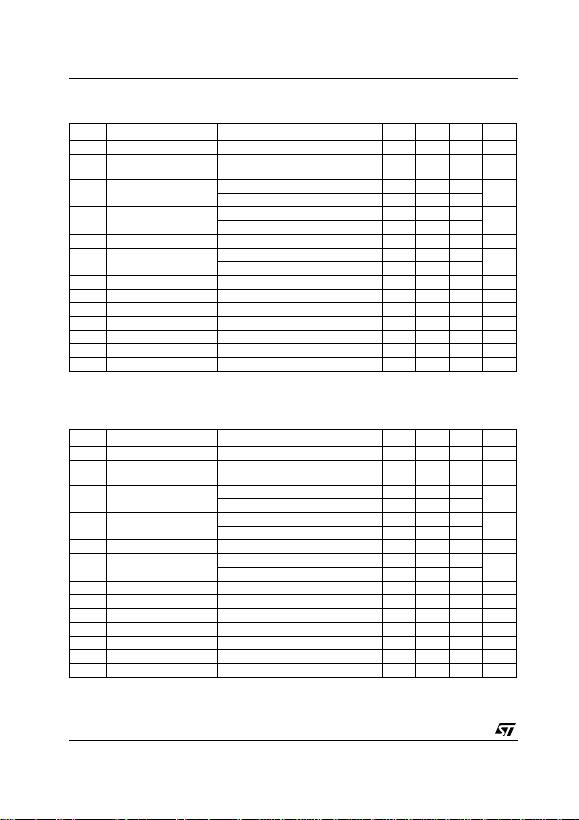L7800 SERIES
10/34
Table 13: Electrical Characteristics Of L785 2C (refer to the test circuits, TJ = 0 to 125°C, V I = 10V ,
IO = 500 mA, CI = 0.33 µF , CO = 0.1 µF unless otherwise specified).
(*) Load and line regulation are specified at constant junction temperature. Changes in VO due to heating effect s must be taken int o acc ount
separately. Pulse te sting with low duty cycle is used.
Table 14: Electrical Characteristics Of L780 6C (refer to the test circuits, TJ = 0 to 125°C, V I = 11V ,
IO = 500 mA, CI = 0.33 µF , CO = 0.1 µF unless otherwise specified).
(*) Load and line regulation are specified at constant junction temperature. Changes in VO due to heating effect s must be taken int o acc ount
separately. Pulse te sting with low duty cycle is used.
Symbol Parameter Test Conditions Min. Typ. Max. Unit
VOOutput Voltage TJ = 25°C 5.0 5.2 5.4 V
VOOutput Voltage IO = 5 mA to 1 A PO 15W
VI = 8 to 20 V 4.95 5.2 5.45 V
VO(*) Line Regulation VI = 7 to 25 V TJ = 25°C 3 105 mV
VI = 8 to 12 V TJ = 25°C 1 52
VO(*) Load Regulation IO = 5 mA to 1.5 A TJ = 25°C 105 mV
IO = 250 to 750 mA TJ = 25°C 52
IdQuiescent Current TJ = 25°C 8 mA
IdQuiescent Current Change IO = 5 mA to 1 A 0.5 mA
VI = 7 to 25 V 1.3
VO/T Output Voltage Drift IO = 5 mA -1 mV/°C
eN Output Noise Voltage B =10Hz to 100KHz TJ = 25°C 42 µV/VO
SVR Supply Voltage Rejection VI = 8 to 18 V f = 120Hz 61 dB
VdDropout Voltage IO = 1 A TJ = 25°C 2 V
ROOutput Resistance f = 1 KHz 17 m
Isc Short Circuit Current VI = 35 V TJ = 25°C 0.75 A
Iscp Short Circuit Peak Current TJ = 25°C 2.2 A
Symbol Parameter Test Conditions Min. Typ. Max. Unit
VOOutput Voltage TJ = 25°C 5.75 6 6.25 V
VOOutput Voltage IO = 5 mA to 1 A PO 15W
VI = 8 to 21 V 5.766.3V
VO(*) Line Regulation VI = 8 to 25 V TJ = 25°C 120 mV
VI = 9 to 13 V TJ = 25°C 60
VO(*) Load Regulation IO = 5 mA to 1.5 A TJ = 25°C 120 mV
IO = 250 to 750 mA TJ = 25°C 60
IdQuiescent Current TJ = 25°C 8 mA
IdQuiescent Current Change IO = 5 mA to 1 A 0.5 mA
VI = 8 to 25 V 1.3
VO/T Output Voltage Drift IO = 5 mA -0.8 mV/°C
eN Output Noise Voltage B =10Hz to 100KHz TJ = 25°C 45 µV/VO
SVR Supply Voltage Rejection VI = 9 to 19 V f = 120Hz 59 dB
VdDropout Voltage IO = 1 A TJ = 25°C 2 V
ROOutput Resistance f = 1 KHz 19 m
Isc Short Circuit Current VI = 35 V TJ = 25°C 0.55 A
Iscp Short Circuit Peak Current TJ = 25°C 2.2 AL7800 SERIES
11/34
Table 15: Electrical Characteristics Of L780 8C (refer to the test circuits, TJ = 0 to 125°C, V I = 14V ,
IO = 500 mA, CI = 0.33 µF , CO = 0.1 µF unless otherwise specified).
(*) Load and line regulation are specified at constant junction temperature. Changes in VO due to heating effect s must be taken int o acc ount
separately. Pulse te sting with low duty cycle is used.
Table 16: Electrical Characteristics Of L788 5C (refer to the test circuits, TJ = 0 to 125°C, V I = 14. 5V ,
IO = 500 mA, CI = 0.33 µF , CO = 0.1 µF unless otherwise specified).
(*) Load and line regulation are specified at constant junction temperature. Changes in VO due to heating effect s must be taken int o acc ount
separately. Pulse te sting with low duty cycle is used.
Symbol Parameter Test Conditions Min. Typ. Max. Unit
VOOutput Voltage TJ = 25°C 7.7 8 8.3 V
VOOutput Voltage IO = 5 mA to 1 A PO 15W
VI = 10.5 to 25 V 7.688.4V
VO(*) Line Regulation VI = 10.5 to 25 V T J = 25°C 160 mV
VI = 11 to 17 V TJ = 25°C 80
VO(*) Load Regulation IO = 5 mA to 1.5 A TJ = 25°C 160 mV
IO = 250 to 750 mA TJ = 25°C 80
IdQuiescent Current TJ = 25°C 8 mA
IdQuiescent Current Change IO = 5 mA to 1 A 0.5 mA
VI = 10.5 to 25 V 1
VO/T Output Voltage Drift IO = 5 mA -0.8 mV/°C
eN Output Noise Voltage B =10Hz to 100KHz TJ = 25°C 52 µV/VO
SVR Supply Voltage Rejection VI = 11.5 to 21.5 V f = 120Hz 56 dB
VdDropout Voltage IO = 1 A TJ = 25°C 2 V
ROOutput Resistance f = 1 KHz 16 m
Isc Short Circuit Current VI = 35 V TJ = 25°C 0.45 A
Iscp Short Circuit Peak Current TJ = 25°C 2.2 A
Symbol Parameter Test Conditions Min. Typ. Max. Unit
VOOutput Voltage TJ = 25°C 8.2 8.5 8.8 V
VOOutput Voltage IO = 5 mA to 1 A PO 15W
VI = 11 to 26 V 8.1 8.5 8.9 V
VO(*) Line Regulation VI = 11 to 27 V TJ = 25°C 160 mV
VI = 11.5 to 17.5 V TJ = 25°C 80
VO(*) Load Regulation IO = 5 mA to 1.5 A TJ = 25°C 160 mV
IO = 250 to 750 mA TJ = 25°C 80
IdQuiescent Current TJ = 25°C 8 mA
IdQuiescent Current Change IO = 5 mA to 1 A 0.5 mA
VI = 11 to 27 V 1
VO/T Output Voltage Drift IO = 5 mA -0.8 mV/°C
eN Output Noise Voltage B =10Hz to 100KHz TJ = 25°C 55 µV/VO
SVR Supply Voltage Rejection VI = 12 to 22 V f = 120Hz 56 dB
VdDropout Voltage IO = 1 A TJ = 25°C 2 V
ROOutput Resistance f = 1 KHz 16 m
Isc Short Circuit Current VI = 35 V TJ = 25°C 0.45 A
Iscp Short Circuit Peak Current TJ = 25°C 2.2 AL7800 SERIES
12/34
Table 17: Electrical Characteristics Of L780 9C (refer to the test circuits, TJ = 0 to 125°C, V I = 15V ,
IO = 500 mA, CI = 0.33 µF , CO = 0.1 µF unless otherwise specified).
(*) Load and line regulation are specified at constant junction temperature. Changes in VO due to heating effect s must be taken int o acc ount
separately. Pulse te sting with low duty cycle is used.
Table 18: Electrical Characteristics Of L781 0C (refer to the test circuits, TJ = 0 to 125°C, V I = 16V ,
IO = 500 mA, CI = 0.33 µF , CO = 0.1 µF unless otherwise specified).
(*) Load and line regulation are specified at constant junction temperature. Changes in VO due to heating effect s must be taken int o acc ount
separately. Pulse te sting with low duty cycle is used.
Symbol Parameter Test Conditions Min. Typ. Max. Unit
VOOutput Voltage TJ = 25°C 8.64 9 9.36 V
VOOutput Voltage IO = 5 mA to 1 A PO 15W
VI = 11.5 to 26 V 8.55 9 9.45 V
VO(*) Line Regulation VI = 11.5 to 26 V TJ = 25°C 180 mV
VI = 12 to 18 V TJ = 25°C 90
VO(*) Load Regulation IO = 5 mA to 1.5 A TJ = 25°C 180 mV
IO = 250 to 750 mA TJ = 25°C 90
IdQuiescent Current TJ = 25°C 8 mA
IdQuiescent Current Change IO = 5 mA to 1 A 0.5 mA
VI = 11.5 to 26 V 1
VO/T Output Voltage Drift IO = 5 mA -1 mV/°C
eN Output Noise Voltage B =10Hz to 100KHz TJ = 25°C 70 µV/VO
SVR Supply Voltage Rejection VI = 12 to 23 V f = 120Hz 55 dB
VdDropout Voltage IO = 1 A TJ = 25°C 2 V
ROOutput Resistance f = 1 KHz 17 m
Isc Short Circuit Current VI = 35 V TJ = 25°C 0.40 A
Iscp Short Circuit Peak Current TJ = 25°C 2.2 A
Symbol Parameter Test Conditions Min. Typ. Max. Unit
VOOutput Voltage TJ = 25°C 9.6 10 1 0.4 V
VOOutput Voltage IO = 5 mA to 1 A PO 15W
VI = 12.5 to 26 V 9.5 10 10.5 V
VO(*) Line Regulation VI = 12.5 to 26 V T J = 25°C 200 mV
VI = 13.5 to 19 V T J = 25°C 100
VO(*) Load Regulation IO = 5 mA to 1.5 A TJ = 25°C 200 mV
IO = 250 to 750 mA TJ = 25°C 100
IdQuiescent Current TJ = 25°C 8 mA
IdQuiescent Current Change IO = 5 mA to 1 A 0.5 mA
VI = 12.5 to 26 V 1
VO/T Output Voltage Drift IO = 5 mA -1 mV/°C
eN Output Noise Voltage B =10Hz to 100KHz TJ = 25°C 70 µV/VO
SVR Supply Voltage Rejection VI = 13 to 23 V f = 120Hz 55 dB
VdDropout Voltage IO = 1 A TJ = 25°C 2 V
ROOutput Resistance f = 1 KHz 17 m
Isc Short Circuit Current VI = 35 V TJ = 25°C 0.40 A
Iscp Short Circuit Peak Current TJ = 25°C 2.2 AL7800 SERIES
13/34
Table 19: Electrical Characteristics Of L781 2C (refer to the test circuits, TJ = 0 to 125°C, V I = 19V ,
IO = 500 mA, CI = 0.33 µF , CO = 0.1 µF unless otherwise specified).
(*) Load and line regulation are specified at constant junction temperature. Changes in VO due to heating effect s must be taken int o acc ount
separately. Pulse te sting with low duty cycle is used.
Table 20: Electrical Characteristics Of L781 5C (refer to the test circuits, TJ = 0 to 125°C, V I = 23V ,
IO = 500 mA, CI = 0.33 µF , CO = 0.1 µF unless otherwise specified).
(*) Load and line regulation are specified at constant junction temperature. Changes in VO due to heating effect s must be taken int o acc ount
separately. Pulse te sting with low duty cycle is used.
Symbol Parameter Test Conditions Min. Typ. Max. Unit
VOOutput Voltage TJ = 25°C 11.5 12 12.5 V
VOOutput Voltage IO = 5 mA to 1 A PO 15W
VI = 14.5 to 27 V 11.4 12 12.6 V
VO(*) Line Regulation VI = 14.5 to 30 V T J = 25°C 240 mV
VI = 16 to 22 V TJ = 25°C 120
VO(*) Load Regulation IO = 5 mA to 1.5 A TJ = 25°C 240 mV
IO = 250 to 750 mA TJ = 25°C 120
IdQuiescent Current TJ = 25°C 8 mA
IdQuiescent Current Change IO = 5 mA to 1 A 0.5 mA
VI = 14.5 to 30 V 1
VO/T Output Voltage Drift IO = 5 mA -1 mV/°C
eN Output Noise Voltage B =10Hz to 100KHz TJ = 25°C 75 µV/VO
SVR Supply Voltage Rejection VI = 15 to 25 V f = 120Hz 55 dB
VdDropout Voltage IO = 1 A TJ = 25°C 2 V
ROOutput Resistance f = 1 KHz 18 m
Isc Short Circuit Current V